0
103

Coding Decoding is one of the most important topics in the CMAT, and also it is an important section. One can utilize this article which consists of the most important questions regarding Coding Decoding. Cracku provides you with the Top 20 very Important Coding Decoding Questions for CMAT based on the questions asked in previous exam papers. Click on the link below to download the Coding Decoding Questions for CMAT PDF with detailed answers.

Question 1: In a certain code language, ‘GUM is coded as ‘49441169’. How will ‘WAX’ be coded as in that language?

a) 5291576

b) 8412525

c) 3611121

d) 2891400

Solution:

G(7) = $7^2$ = 49
U(21) = $(21)^2$ = 441
M(13) = $(13)^2$ = 169
Similarly,
W(23) = $(23)^2$ = 529
A(1) = $(1)^2$ = 1
X(24) = $(24)^2$ = 576
‘WAX’ is coded as ‘5291576’.
$\therefore$ The correct answer is option A.

Question 2: In a certain code language, ‘TAPE’ is written as ‘SUZBOQDF’. How will ‘MOCK’ be written as in that language?

a) MOPQDELM

b) LNNPBDJL

c) LOPQBCKL

d) KNNPBEBL

Solution: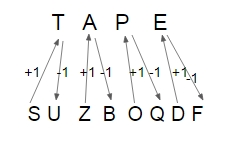Similarly,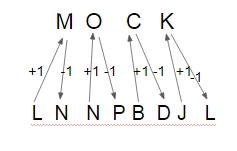$\therefore$ The correct answer is option B.

Question 3: In certain code language, ‘FOX’ is coded as ‘108’ and ‘SOUP’ is coded as ‘148’. How will ‘UPSIDE’ be coded as in that language?

a) 528

b) 590

c) 385

d) 494

Solution:

For the ‘FOX’,
[(27 – 6) + (27 – 15) + (27 – 24)] $\times$ number of letter
= (21 + 12 + 3) $\times$ 3
= 36 $\times$ 3 = 108
For the ‘Soup’,
[(27 – 19) + (27 – 15) + (27 – 21) + (27 – 16)] $\times$ number of letter
= (8 + 12 + 6 + 11) $\times$ 4
= 37 $\times$ 4 = 148
Similarly,
For the ‘UPSIDE’,
[(27 – 21) + (27 – 16) + (27 – 19) + (27 – 9) + (27 – 4) + (27 – 5)] $\times$ number of letter
= (6 + 11 + 8 + 18 + 23 + 22) $\times$ 6
= 88 $\times$ 6 = 528
$\therefore$ The correct answer is option A.

Question 4: Ina certain code language, ‘LAMP’is coded as ‘2422632’. Howwill ‘FORK’be codedas in that language?

a) 12302624

b) 12303622

c) 12303424

d) 12303422

Solution:

‘LAMP’ is coded as ‘2422632’,
L $\rightarrow$ 12 $\times$ 2 = 24
A $\rightarrow$ 1 $\times$ 2 = 2
M $\rightarrow$ 13 $\times$ 2 = 26
P $\rightarrow$ 16 $\times$ 2 = 32
Similarly,
F $\rightarrow$ 6 $\times$ 2 = 12
O $\rightarrow$ 15 $\times$ 2 = 30
R $\rightarrow$ 18 $\times$ 2 = 16
K $\rightarrow$ 11 $\times$ 2 = 22
‘FORK’ is coded as ‘12301622’.
$\therefore$ The correct answer is option B.

Question 5: In a certain code language, ‘FIGURE’ is written as ‘DJEVPF’. Howwill ‘DECODE’ be written as in that language ?

a) BFDNBF

b) BDANBD

c) BDDNBD

d) BFAPBF

Solution:

‘FIGURE’is written as ‘DJEVPE’,
F – 2 = D
I + 1 = J
G – 2 = E
U + 1 = V
R – 2 = P
E + 1 = F
Similarly,
D – 2 = B
E + 1 = F
C – 2 = A
O + 1 = P
D – 2 = B
E + 1 = F
‘DECODE’ is written as ‘BFAPBF’.
$\therefore$ The correct answer is option D.

Question 6: Ina certain code language, ‘DEPEND’ is written as ‘EPHTJJ’. How will ‘TRAVEL’ be written as in that language?

a) MGWEWY

b) NGYFWZ

c) MGYEWZ

d) MGZEXZ

Solution: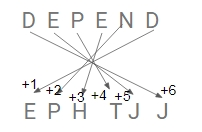Similarly,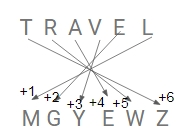$\therefore$ The correct answer is option C.

Question 7: In a certain code language, ‘SERVANT’ is coded as ‘195182211420’. How will ‘MAGNIFY’ be coded asin that language?

a) 1426693625

b) 1316143522

c) 1317149625

d) 1417139625

Solution:

Word coded according to the alphabet number so,

‘MAGNIFY’ is coded as ‘1317149625’.
$\therefore$ The correct answer is option C.

Question 8: Ina certain code language, ‘BLINK’is coded as ’78’ and ‘”PURSE’is coded as ’54’. How will ‘FIRST’be coded asin that language?

a) 63

b) 71

c) 64

d) 76

Solution:

B L I N K = (27 – 2) + (27 – 12) +(27 – 9) + (27 – 14) + (27 – 11) = 25 + 15 + 18 + 13 + 16 = 87

P U R S E = (27 – 16) + (27 – 21) +(27 – 18) + (27 – 19) + (27 – 5) = 11 + 6 + 9 + 8 + 22 = 56

Similarly,

F I R S T = (27 – 6) + (27 – 9) +(27 – 18) + (27 – 19) + (27 – 20 )= 21 + 18 + 9 + 8 + 7 = 63
$\therefore$ The correct answer is option A.

Question 9: Ina certain code language, ‘NECTAR’is written as ‘DFOSBU’. How will ‘CARPET’be written as in that language?

a) SBDUFQ

b) DBSQGR

c) SBDVFR

d) BDSSUG

Solution: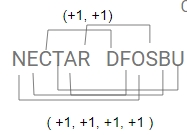Similarly,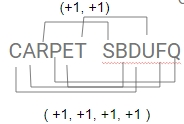$\therefore$ The correct answer is option A.

Question 10: In a certain code language, ‘PAINTS’is written as ‘CRPKUV’. How will ‘”PURITY’ be written as in that language?

a) WRKTAV

b) XSISBM

c) WRKSNU

d) VSKTAZ

Solution: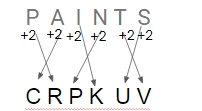Similarly,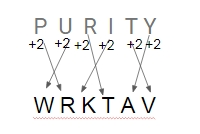$\therefore$ The correct answer is option A.

Question 11: Ina certain code language, ‘SOLID’is coded as ’64’ and ‘POUR’is coded as ’74’. How will ‘TON’ be coded as in that language?

a) 49

b) 60

c) 85

d) 52

Solution:

SOLID = (19 + 1) + (15 + 1) + (12 + 1) + (9 + 1) + (4 + 1) = 64
POUR = (16 + 1) + (15 + 1) + (21 + 1) + (18 + 1) = 74
Similarly,
TON = (20 + 1) + (15 + 1) + (14 + 1) = 52
$\therefore$ The correct answer is option D.

Question 12: In a certain code language, ‘VERBAL’ is written as ‘QNMYXK’ and ‘REACH?’is written as ‘MNXZF’. How will ‘BRAVE’ be written as in that language?

a) KQNMX

b) YNXQM

c) YMXQN

d) KMQXN

Solution:

V is written as Q,
E is written as N,
R is written as M,
B is written as Y,
A is written as X,
L is written as K,

And
R is written as M,
E is written as N,
A is written as X,
C is written as Z,
H is written as F,
Similarly,
‘BRAVE’ is written as ‘YMXQN’
$\therefore$ The correct answer is option C.

Question 13: In a certain code language, BREATHER is coded as 29512859. How will AVIATION be coded as in that language?

a) 14912965

b) 14912956

c) 12912954

d) 12910965

Solution:

B = 2
R(18) = 1 + 8 = 9
E = 5
A = 1
T(20) = 2 + 0 = 2
H = 8
E = 5
R(18) = 1 + 8 = 9
Similarly,
A = 1
V(22) = 2 + 2 = 4
I = 9
A = 1
T(20) = 2 + 0 = 2
I = 9
O(15) = 1 + 5 = 6
N(14) = 1 + 4 = 5
‘AVIATION’  is coded as ‘14912965’.
$\therefore$ The correct answer is option A.

Question 14: In a certain code language, ‘FIXATION’ is written as ‘AXIFNOIT’. How will ‘GLYCERIN’ be written as in that language?

a) CYLGNIRE

b) NIRECYLG

c) LGZDGINR

d) YLGECRNI

Solution: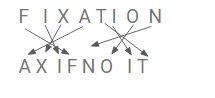Similarly,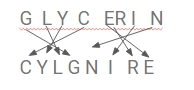$\therefore$ The correct answer is option A.

Question 15: In a certain code language, ‘SPECIAL’ is coded as ‘20176410213’. How will ‘MACHINE’ be coded as in that language?

a) 1324810155

b) 1424901056

c) 1424910156

d) 1524910146

Solution:

‘SPECIAL’ is coded as ‘20176410213’.
Like as,
S + 1 = 20
P + 1 = 17
E + 1 = 6
C + 1 = 4
I + 1 = 10
A + 1 = 2
L + 1 = 13
Similarly,
For ‘MACHINE’,
(M + 1)(A + 1)(C + 1)(H + 1)(I + 1)(N + 1)(E + 1)
= 1424910156
$\therefore$ The correct answer is option C.